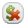# Cost CalculationsUpdated 6 months ago by Leigh Hutchens

Quinyx is able to calculate salary costs. This is not linked to any salary payment, it is merely used for calculating cost. The following data must be in place for cost calculation to work.

1. Enter hourly pay – either an average pay in agreement templates and/or the actual hourly pay for the individual agreements
Time > Agreement templates - Agreement or Employee > Agreement/Accessibility
2. Set a value for all salary types to be included in the calculation
Time > Tables > Salary types
(  If a salary type should not generate a cost, select 0% a hourly salary. )
3. Enter social costs in agreement templates
Time > Agreement templates - Agreement
4. To include costs for unmanned shifts, cost must be defined for the shift type, both hourly pay and value of any unsocial hours. Social costs are not included here, so they have to be added.
5. Calculation of salary cost is enabled on the unit card. Here, it is also possible to select whether Quinyx is to calculate cost automatically every time the user accesses Schedule and Time
Settings > Unit > Daily summaryIf you deselect automatic cost calculation, the cost can easily be calculated by clicking this symbol at the top right of the screen in Schedule/Time views.You can view salary costs under the tab Time, Scheduled Staff and in the daily views.

Note -It is possible to hide the costs in these views for relevant employees, e.g. local or section managers, if necessary. To do that -  delete the access right Costs under Time - Time, Schedule - Schedules, Schedule - Scheduled staff.

### How is Cost Calculated in Quinyx?

There are three different types of costs:

• Net cost (cost from salary transactions)
• Leave compensation (percentage of net costs)
• Social costs (percentage of both net cost and leave compensation combined)

### Calculation example

So, let's say an employee is scheduled to work 160 hours, all of which will generate base salary at \$10 per hour. They have a leave compensation of 12% and social costs of 25%.

• Calculate base salary → 160 hours x \$10 = \$1600
• Calculate leave compensation → \$1600 x 12% = \$192
• Calculate social costs → (\$1600 + \$192) x 25% = \$448
• Total cost = \$1600 + \$192 + \$448 = \$2240
• Or a quicker way of calculating total cost =  ((1600 x \$10) x 1.12 ) x 1.25 = \$2240